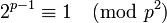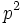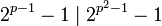# Square of Wieferich prime is Poulet number

(diff) ← Older revision | Latest revision (diff) | Newer revision → (diff)

## Statement

Supposeis a Wieferich prime, i.e., a prime number such that:Then,is a Poulet number (also called Sarrus number), i.e., a Fermat pseudoprime to base 2.

## Proof

Given:is a prime such thatTo prove:Proof: We have:Thus,divides. This gives that:Combining this with the given information, we get that.